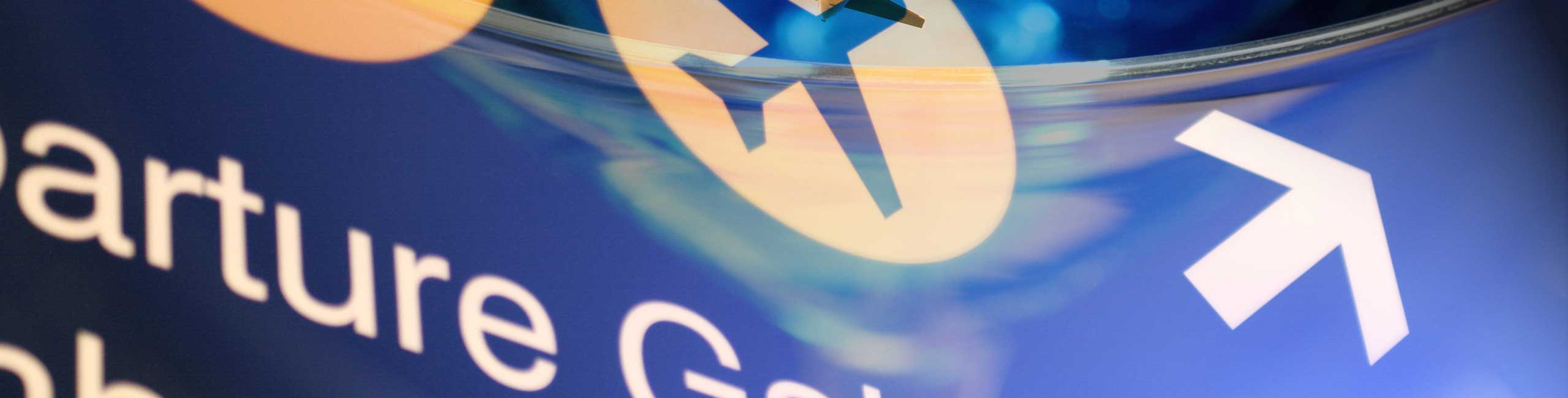Reflection of Light Rays, Examples and Solutions

Reflection of Light Rays on a Reflecting Surface

When a light ray strikes a surface separating two media with different optical properties, part of the light energy is reflected back to the media where it coming from. When light strikes a perfectly reflecting surface, such a mirror for example, all the light energy is reflected.
We first look at the vocabulary used to describe the reflection of light.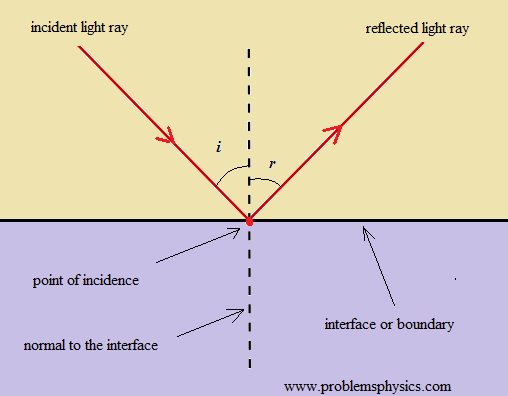The surface separating the two media is called the interface or boundary.
The point at which the incident ray strikes the interface is called the point of incidence.
i is the angle made by the incident ray and the normal to the interface and is called the angle of incidence.
r is the angle made by the reflected ray and the normal to the interface and is called the angle of reflection.

Laws of reflection
(1) The incident light ray, the reflected light ray and the normal to the interface at the point of incidence make a plane called the plane of incidence
(2) The angle of incidence and the angle of reflection have the same size.

Example 1: A light ray strikes a reflective plane surface at an angle of 56� with the surface.
a) Find the angle of incidence.
b) Find the angle of reflection.
c) Find the angle made by the reflected ray and the surface.
d) Find the angle made by the incident and reflected rays.

 Solution: We use the diagram shown below to answer the questions. a) angle of incidence: i = 90 - 56 = 34 � b) angle of reflection r = i = 34 � (by the law of reflection) c) q = 90 - r = 90 - 34 = 56 � d) i + r = 34 + 34 = 68 �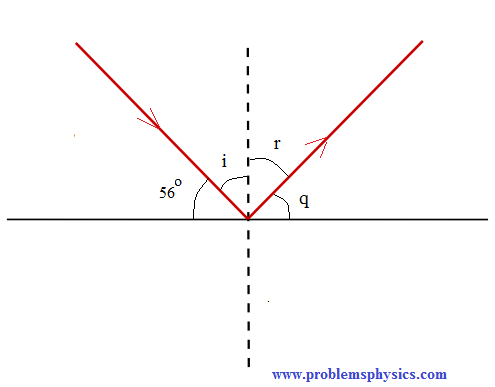Example 2: A ray of light is reflected by two parallel mirrors (1) and (2) at points A and B. The ray makes an angle of 25� with the axis of the two mirrors.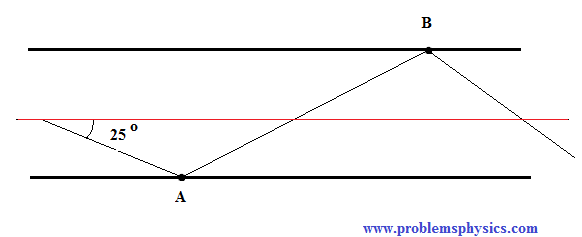a) What is the angle of reflection at the point of incidence A?
b) What is the angle of reflection at the point of incidence B?
c) If the distance between the two mirrors id d = 4 cm and the length L of the two mirror system is 3 meters, approximately how many reflections take place between the two mirrors?
d) In a real system, at each reflection, there are losses of the light energy travelling between the two mirrors. If L and d are fixed, what can be done to decrease the number of reflections on the mirrors and hence the energy lost by reflection?

 Solution: Let's use the diagram shown below to answer the questions. n1 and n2 are normal to the reflecting mirrors and are therefore perpendicular to the axis of the two mirror system.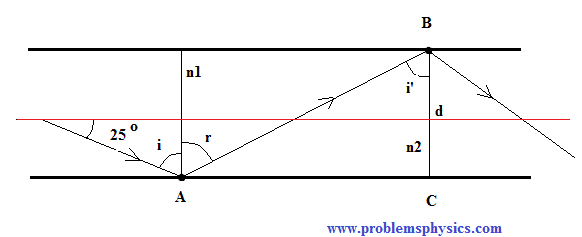a) i = 90 - 25 = 75 � b) the two mirrors are parallel and AB intersect both of them, hence angle r and i' are alternate interior and therefore equal in size. Also r = i (law of reflection). Hence i' = r = i = 75 � c) If the distance between the two mirrors id d = 4 cm, AC can be calculated as follows tan(75�) = AC / d = AC / 4 AC = 4 tan(75�) = 15 cm The number of reflections N that will take place between the two mirrors may be approximated as follows. N = L / AC = 3 m / 15 cm = 20 d) Let the angle made by the light ray and the axis of the two mirror system be α instead of 25 �. The angle of incidence i = 90 - α = r = i' tan(i') = tan( 90 - α) = cot (α) = AC / d AC = d cot(α) number of reflections = N = L / AC = L / d cot(α) If L and d are fixed, the only way to decrease N is to increase cot(α) and hence decrease α.

Example 3: A light ray at an angle of incidence i first strikes mirror (1) at point A, then mirror (2) at point B and then mirror (1) again at point C. The two mirrors make an angle α.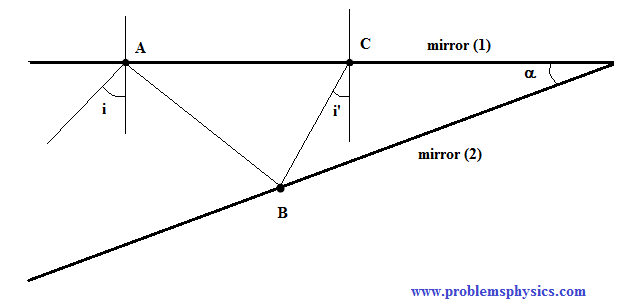Express the angles of incidence at point B and point C in terms of the angle of incidence i and angle α?

 Solution: We first complete the given diagram drawing the various rays at points of incidence. Diagram shown below.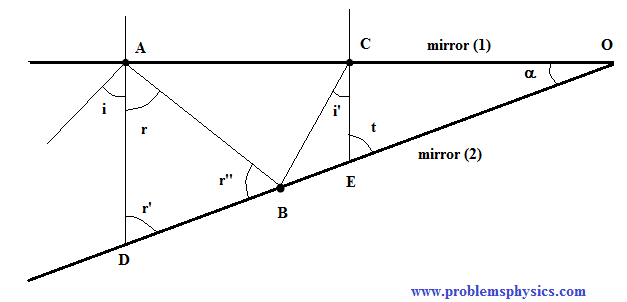Let AD and CE be the normals to mirror(1) at A and C respectively. r = i (law of reflection) In triangle OAD, we can write α + 90 + r' = 180 � Simplify to get: r' = 90 - α In triangle ADB, we have r + r' + r" = 180 � r" = 180 - r' - r Substitute r' by 90 - α and r by i in r" to obtain r" = 90 + α - i The angle of incidence at B is given by 90 - r" = 90 - (90 + α - i) = i - α In triangle CBE, we have ∠CBE = r" (incident ray and reflected ray make angles with surface of reflection of equal size) In triangle OCB, we have α + 90 + i' + ∠CBE = 180 � Substitute ∠CBE by r" α + 90 + i' + r" = 180 � Substitute r" by 90 + α - i to obtain α + 90 + i' + 90 + α - i = 180 � Simplify to obtain the angle of incidence i' at C i' = i - 2 α At each reflection, the angle of incidence decreases by an amount equal to the size of angle α.

Example 4: 4) Find angle α made by the system of the two mirrors shown in the figure below so that the incident ray at A and the reflected ray at B are parallel.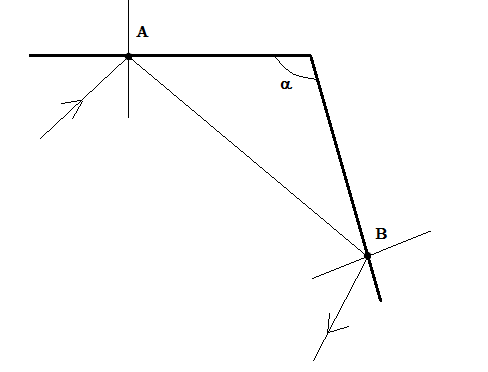Solution: We first complete the given diagram with the angles of incidence and reflection as shown below and also labeling the incident and reflected rays.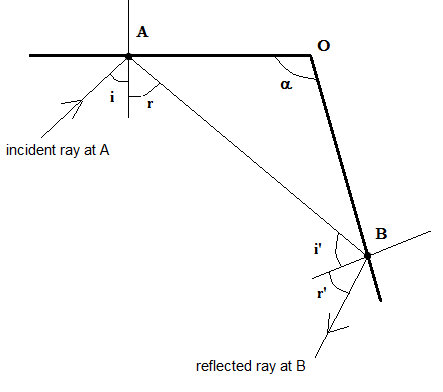For the incident ray at A and the reflected ray at B to be parallel, angles i + r and i' + r' have to be supplementary.(geometry: parallel lines cut by a transversal). Hence i + r + i'+ r' = 180 � by law of reflection : r = i and r' = i' Substitute to obtain i + i + i' + i' = 180 � i + i' = 90 In triangle AOB, we have α + (90 - r) + (90 - i') = 180 � α = r + i' = i + i' = 90 � If α = 90 �, the incident ray at A and the reflected ray at B are parallel.